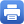Columbia Home
Probability and Financial Mathematics

## OverviewProbability is concerned with the mathematical analysis of random phenomena. It is younger than most other areas of Mathematics, as it originated with the study of games of chance by Pascal and Fermat (1654); since then it has become indispensable for dealing with randomness in almost all branches of science. Today, in addition to its strong position as a full-fledged area within mathematics — and its interactions with analysis, partial differential equations, geometry, combinatorics, number theory and mathematical physics — probability forms the language of statistics and of the quantitative social sciences. It is essential in the discovery and study of macroscopic regularities that occur when large systems of particles, organisms, or agents, interact according to the laws of physics, biology, or economics; in the study of population genetics and of the genome; in the study of how signals are transmitted through a noisy channel, and then recovered; in the design and analysis of large-scale communication, neural or queueing networks, and of algorithms for combinatorial optimization, computerized tomography, signal processing, pattern recognition, and so on. A central role in several of these applications is played by the development of stochastic analysis over the last 70 years, starting with the pioneering work of K. Ito in 1942.

The Columbia probability group has a strong interest in several areas of probability theory, including stochastic control, stochastic games, and statistical mechanics and mathematical physics.

## Mathematical Finance

Probability has also been central in the study of finance, ever since Bachelier pioneered in 1900 the mathematical study of Brownian motion and understood its significance as a tool for the analysis of financial markets (five years before Einstein developed his physical theory of Brownian motion). The theory of finance, brought to worldwide attention with the award of the Nobel prize in economics to Markowitz, Sharpe, Miller and then to Merton and Scholes, tries to understand how financial markets work, how they can be made more efficient, how they should be regulated, and how they can help manage the risk inherent in various economic activities. Over the last 30 years this theory has become increasingly mathematical, to the extent that problems arising in finance are now not just drawing upon, but are also driving research in stochastic analysis, partial differential equations, and control theory. At the same time, the development of sophisticated analytical and numerical methods, often based on partial differential equations and on their numerical solution, has helped to increase the relevance of these developments in the everyday practice of finance.

## Statistical Mechanics and Mathematical Physics

There is a long history of fruitful exchanges between Probability Theory and Statistical Mechanics and Mathematical Physics. Models of physical systems present challenging and relevant problems to probabilists; ideas and techniques flow back and forth. A general problem is to understand how microscopic noise and local interactions generate random structures on the macroscopic scale, and to describe qualitatively and quantitatively these structures. While the formulation of basic models of physical phenomena, such as percolation, the Ising model, random growth models and interacting particle systems, is completely elementary, their analysis involves a wide array of techniques and concepts: combinatorics and graphs, complex analysis and algebraic curves, operators and representation theory, symmetric functions and integrable systems, Brownian motion, Gaussian fields, stochastic partial differential equations… The fact that current progress toward the understanding of these models involves such diverse ideas is a testimony to the vivacity and depth of the field.

## People

Ivan Corwin
Professor
Ph.D. Courant Institute of Mathematical Sciences, NYU, 2011.
Interests: Probability, Mathematical/Statistical Physics, Integrable Systems, Symmetric Function Theory, Random Growth Models, Interacting Particle Systems, Directed Polymers, Stochastic Partial Differential Equations, Random Matrix Theory

Julien Dubedat
Professor
Ph.D.  Ecole Normale Superieure and Universite Paris-Sud (Orsay), 2004
Interests: Statistical mechanics, Schramm-Loewner Evolutions, Gaussian Free Field, lattice models.

Ioannis Karatzas
Eugene Higgins Professor of Applied Probability
Ph.D. Columbia University, 1980
Interests: Probability and Mathematical Statistics, Random Processes, Stochastic Analysis, Optimization, Mathematical Economics and Finance.

Lars Tyge Nielsen
Senior Lecturer / Director of the Mathematics of Finance MA Program
Ph.D. Harvard University, 1985
Interests: Mathematical Finance

Marcel Nutz
Professor, joint with Statistics department
Ph.D.  ETH Zurich, 2010.
Interests: Probability Theory, Stochastic Optimal Control, Mathematical Finance

## Friends of Probability at Columbia

Richard Davis (Statistics Department)
Igor Krichever (Mathematics Department)
Andrei Okounkov (Mathematics Department)
Victor de la Pena (Statistics Department)
Philip Protter (Statistics Department)
Bodhisattva Sen (Statistics Department)
Michael Weinstein (Applied Mathematics, and Mathematics)

## Regular Activities

Minerva Lectures: These special lecture series in probability and mathematical finance are made possible by the generous support of the Minerva Research Foundation.
Time and location vary by semester.

Columbia-Princeton Probability Day (Spring): Annual one-day conference alternating between Columbia and Princeton.

Columbia-Courant Joint Probability Seminar: Periodic half-day joint seminar alternating between Columbia and Courant, NYU.

Northeast Probability Seminar (Fall): Annual two-day conference organized jointly by Columbia, CUNY and NYU.

## Probability in NYCPrint this page